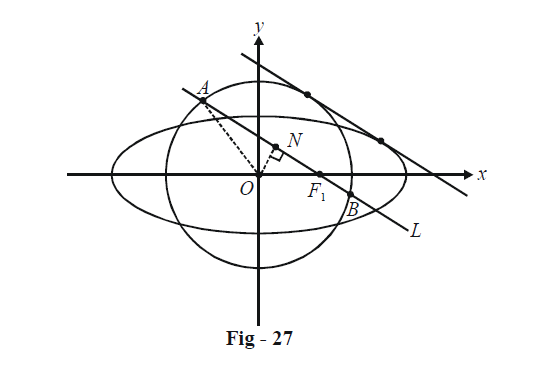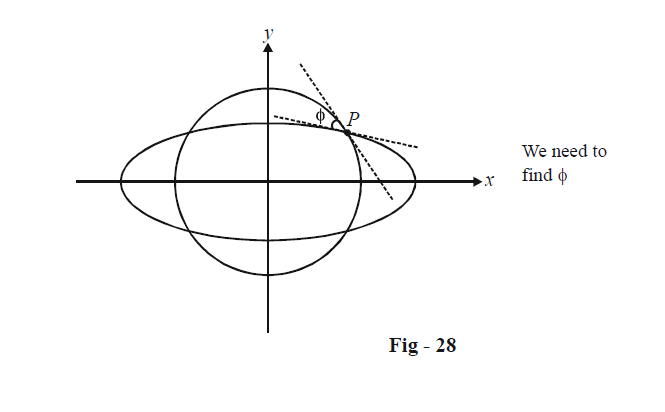# Examples On Tangents To Ellipses Set-5

Go back to  'Ellipse'

Example – 24

A straight line L touches the ellipse $$\frac{{{x^2}}}{{{a^2}}} + \frac{{{y^2}}}{{{b^2}}} = 1$$ and the circle $${x^2} + {y^2} = {r^2}$$ (where b < r < a). A focal chord of the ellipse parallel to L meets the circle in A and B. Find the length of AB.

Solution: L is a common tangent to the ellipse and the circle. We can assume the equation of L to be (using the form of an arbitrary tangent to an ellipse) :

$y = mx + \sqrt {{a^2}{m^2} + {b^2}}$Since L is a tangent to the circle too, its distance from (0, 0) must equal r. Thus,

\begin{align}&\frac{{\sqrt {{a^2}{m^2} + {b^2}} }}{{\sqrt {1 + {m^2}} }} = r\\ & \Rightarrow \quad m = \pm \sqrt {\frac{{{r^2} - {b^2}}}{{{a^2} - {r^2}}}}\qquad\qquad\quad ...\left( 1 \right)\end{align}

The equation of AB( which passes through $${F_1}(ae,\,\,0))$$ can now be written as

\begin{align}&y - 0 = m(x - ae)\\ &\Rightarrow \quad mx - y = mae\quad\quad\quad...\left( 2 \right)\end{align}

To evaluate the length AB, one alternative is to find the intercept that the circle $${x^2} + {y^2} = {r^2}$$ makes on the line AB given by (2).

However, a speedier approach would be to use the Pythagoras theorem in $$\Delta OAN.$$ OA is simply the radius r. ON is the perpendicular distance of O from the line given by (2). Thus,

$ON = \frac{{mae}}{{\sqrt {{m^2} + 1} }}$

Finally, we have

\begin{align}&\; \;AB=2AN \\ & \qquad=2\sqrt{O{{A}^{2}}-O{{N}^{2}}} \\ &\qquad =2\sqrt{{{r}^{2}}-\frac{{{m}^{2}}{{a}^{2}}{{e}^{2}}}{{{m}^{2}}+1}} \\ &\qquad =2\sqrt{{{r}^{2}}-\left( \frac{{{r}^{2}}-{{b}^{2}}}{{{a}^{2}}-{{b}^{2}}} \right)\frac{{{a}^{2}}{{e}^{2}}({{a}^{2}}-{{r}^{2}})}{({{a}^{2}}-{{b}^{2}})}} \\ & \qquad=2b \\\end{align}

The length of the chord AB is equal to 2b, the same as the minor axis of the ellipse.

Example - 25

Find the angle of intersection of the ellipse $$\frac{{{x^2}}}{{{a^2}}} + \frac{{{y^2}}}{{{b^2}}} = 1$$ and the circle $${x^2} + {y^2} = ab.$$

Solution: The semi-major and semi-minor axis of the ellipse are of lengths a and b respectively whereas the radius of the circle is $$\sqrt {ab} .$$ Note that

$b < \sqrt {ab} < a$

Thus, the circle will intersect (symmetrically) the ellipse in four points.Consider any point of intersection, say P, the one in the first quadrant. The coordinates of P can be assumed to be $$(a\cos \theta ,\,b\sin \theta ).$$ Since P also lies on the circle, we have

\begin{align}&{a^2}{\cos ^2}\theta + {b^2}{\sin ^2}\theta = ab\\& \Rightarrow\quad ({a^2} - {b^2}){\cos ^2}\theta = b(a - b)\\&\left. \begin{array}{l} \Rightarrow & {\cos ^2}\theta = \frac{b}{{a + b}}\\ \Rightarrow & {\sin ^2}\theta = \frac{a}{{a + b}}\end{array} \right\}\qquad\qquad\quad\quad...\left( 1 \right)\end{align}

At P, the tangent to the ellipse has the slope

${{m}_{E}}=\frac{-b}{a}\cot \theta =-{{\left( \frac{b}{a} \right)}^{{}^{3}\!\!\diagup\!\!{}_{2}\;}}$

while the tangent to the circle has the slope

${{m}_{C}}=\frac{-a}{b}\cot \theta =-{{\left( \frac{a}{b} \right)}^{{}^{1}\!\!\diagup\!\!{}_{2}\;}}~\left( Again,\text{ }using\text{ }\left( 1 \right) \right)$

Thus, the angle of intersection is given by

\begin{align} & \tan \phi =\left| \frac{{{m}_{C}}-{{m}_{E}}}{1+{{m}_{C}}{{m}_{E}}} \right|=\left| \frac{{{\left( \frac{a}{b} \right)}^{{}^{1}\!\!\diagup\!\!{}_{2}\;}}-{{\left( \frac{b}{a} \right)}^{{}^{3}\!\!\diagup\!\!{}_{2}\;}}}{1+\frac{b}{a}} \right|=\frac{a+b}{\sqrt{ab}} \\ & \Rightarrow\; \phi ={{\tan }^{-1}}\left( \frac{a+b}{\sqrt{ab}} \right) \\ \end{align}

## TRY YOURSELF - II

Q. 1 Prove that the tangents at the extremities of the latus rectum of an ellipse intersect on the corresponding directrix.

Q. 2 Let P and Q be points on the ellipse $$\frac{{{x^2}}}{{{a^2}}} + \frac{{{y^2}}}{{{b^2}}} = 1$$ whose eccentric angles differ by $$\frac{\pi }{2}.$$ Tangents at P and Q intersect at R. What is the locus of R?

Q. 3 Prove that the locus of the mid-point of the portion of the tangent to the ellipse $$\frac{{{x^2}}}{{{a^2}}} + \frac{{{y^2}}}{{{b^2}}} = 1$$ intercepted between the coordinate axes is $${b^2}{x^2} + {a^2}{y^2} = 4{x^2}{y^2}.$$

Q. 4 We generalise Question - 2 (above) in this question. What is the locus of the point of intersection of tangents to the ellipse $$\frac{{{x^2}}}{{{a^2}}} + \frac{{{y^2}}}{{{b^2}}} = 1$$ at points whose eccentric angles differ by a constant $$2\alpha$$ .

Q. 5 Find the locus of the foot of perpendicular on any tangent to the ellipse $$\frac{{{x^2}}}{{{a^2}}} + \frac{{{y^2}}}{{{b^2}}} = 1$$ from either of its foci.

Q. 6 A family of ellipses have the same major axis, but different minor axis. Prove that the tangents at the end-points of their latus - rectums will always pass through a fixed point.

Q. 7 Let P be any point on the ellipse $$\frac{{{x^2}}}{{{a^2}}} + \frac{{{y^2}}}{{{b^2}}} = 1$$ with y-coordinate k. Prove that the angle between the tangent at P and the focal chord through P is $${\tan ^{ - 1}}\left( {\frac{{{b^2}}}{{aek}}} \right).$$

Q. 8 A tangent to $$\frac{{{x^2}}}{{{a^2}}} + \frac{{{y^2}}}{{{b^2}}} = 1$$ cuts the axes in A and B and touches the ellipse at P in the first quadrant. What is the equation of this tangent if AP = PB ?

Q. 9 If the tangent at any point on $$\frac{{{x^2}}}{{{a^2}}} + \frac{{{y^2}}}{{{b^2}}} = 1$$ makes an angle $$\alpha$$ with the major axis and an angle $$\beta$$ with the focal radius of the point of contact, show that the eccentricity of the ellipse e satisfies \begin{align}e = \frac{{\cos \beta }}{{\cos \alpha }}.\end{align}

Q. 10 Prove that the tangent at any point on the ellipse bisects the external angle between the focal radii of that point.